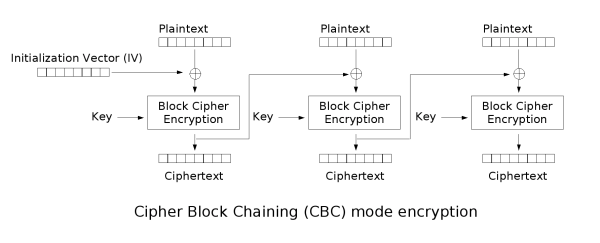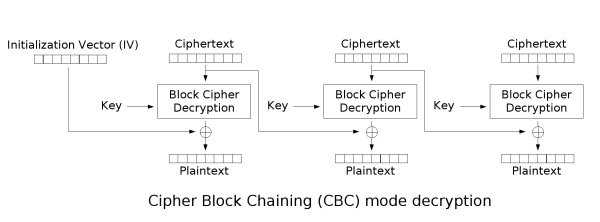# CBC¶

CBC is called the Cipher-block chaining mode, here

• IV does not require confidentiality
• IV must be unpredictable and must be complete.

## Encryption¶## decryption¶1. The ciphertext block is not only related to the current ciphertext block, but also related to the previous ciphertext block or IV, hiding the statistical properties of the plaintext.
2. Has a limited two-step error propagation feature, that is, a one-bit change in the ciphertext block only affects the current ciphertext block and the next ciphertext block.
3. With self-synchronization feature, that is, the k-th block is correct, the k+1th block can be decrypted normally.

1. Encryption cannot be parallel, decryption can be parallel.

## Application¶

CBC is widely used

• Common data encryption and TLS encryption.
• Integrity and identity authentication.

## Attack¶

### Byte reversal attack¶

#### Principle¶

The principle of byte inversion is very simple, we observe the decryption process can find the following characteristics:

• IV vector affects the first plaintext grouping
• The nth ciphertext packet can affect the n + 1 plaintext packet

Assuming that the $n$ ciphertext is grouped as $C_n$, the decrypted $n$ plaintext is grouped as $P_n$.

Then $P_{n+1}=C_n~\text{xor}~f(C_{n+1})$.

The $f$ function is $\text{Block Cipher Decryption}$ in the figure.

For the original text and ciphertext of a certain information, then we can modify the $n$ ciphertext block $C_n$ to $C_n~\text{xor}~P_{n+1}~\text{xor}~ A$. Then decrypt the ciphertext, then the decrypted $n$ plaintext will soon become $A$.

#### Example¶

from flag import FLAG

from Crypto.Cipher import AES

from Crypto import Random

import base64

BLOCK_SIZE=16

pad = lambda s: s + (BLOCK_SIZE - len(s) % BLOCK_SIZE) * chr(BLOCK_SIZE - len(s) % BLOCK_SIZE)

unpad = lambda s: s [: - ord (s [len (s) - 1:])]

prefix = "flag="+FLAG+"&userdata="

suffix = "&user=guest"

print "1. encrypt"

print "2. decrypt"

return raw_input("> ")

def encrypt():

plain = prefix+data+suffix

aes = AES.new(passphrase, AES.MODE_CBC, IV)

def decrypt():

data = raw_input("input data: ")

aes = AES.new(passphrase, AES.MODE_CBC, IV)

plain = unpad (aes.decrypt (base64.b64decode (data)))
print 'DEBUG ====> ' + plain

print plain

else:

def main():

for _ in range(10):

if cmd=="1":

encrypt()

elif cmd == &quot;2&quot;:
decrypt()

else:

exit()

if __name__=="__main__":

main()


Visible topic I hope we provide an encrypted string, if the final content of this string is admin. The program will output clear text. Therefore, the problem flow is to provide a plain text first, and then modify the ciphertext so that the final content of the decrypted string is admin. We can enumerate the length of the flag to determine where we need to modify it.

The following is exp.py

from pwn import *

import base64

for x in range(10, 100):

r = remote('xxx.xxx.xxx.xxx', 10004)

#r = process('./chall.sh')

r.sendlineafter('> ', '1')

cipher = list(base64.b64decode(r.recv()))

#print 'cipher ===>', ''.join(cipher)

BLOCK_SIZE = 16

prefix = "flag=" + 'a' * x + "&userdata="
suffix = "&user=guest"

plain = prefix + data + suffix

idx = (22 + x + pad) % BLOCK_SIZE + ((22 + x + pad) / BLOCK_SIZE - 1) * BLOCK_SIZE

cipher[idx + 0] = chr(ord(cipher[idx + 0]) ^ ord('g') ^ ord('a'))

cipher[idx + 1] = chr(ord(cipher[idx + 1]) ^ ord('u') ^ ord('d'))

cipher[idx + 2] = chr(ord(cipher[idx + 2]) ^ ord('e') ^ ord('m'))

cipher[idx + 3] = chr(ord(cipher[idx + 3]) ^ ord('s') ^ ord('i'))

cipher[idx + 4] = chr(ord(cipher[idx + 4]) ^ ord('t') ^ ord('n'))

r.sendlineafter('> ', '2')

r.sendlineafter('input data: ', base64.b64encode(''.join(cipher)))

msg = r.recvline()

if 'you are not admin' not in msg:

print msg

break

r.close()